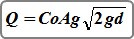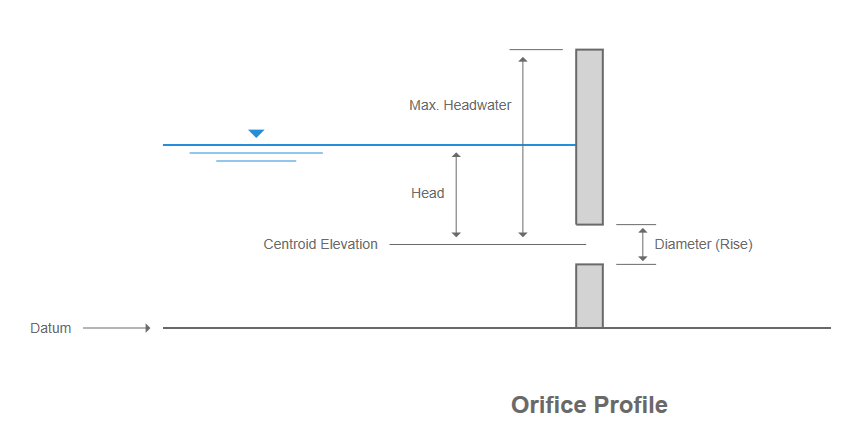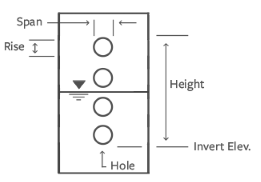# Orifices

## Circular & Rectangular

Studio Express uses the standard orifice equation for Circular and Rectangular orifices.Where:
Co = 0.60 (or as set in Settings)
Ag = clear opening area in sqft (sqm)
g = 32.16 (9.8) gravity
d = head as measured from the centroid in ft (m)

##Orifice Plates

This is a special kind of orifice structure as shown below which contains a series of same-sized holes all within a vertical height.The following formula by McEnroe, 1988 is used to estimate the outflow.Where:
Q = discharge (cfs)
Cp = 0.61
A = cross-sectional area of all the holes (sqft)
Hs = height (ft)
H = distance between water surface and the Invert Elevation (ft)

Updated on May 9, 2018